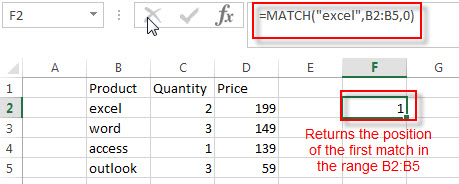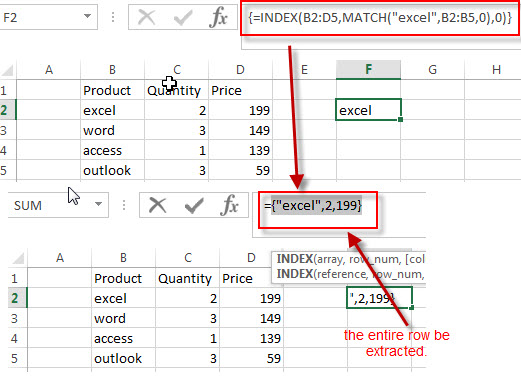# How to Lookup Entire Row using INDEX/MATCH

This post explains that how to lookup entire row and return values of the entire row in a table or range in excel.

## Lookup Entire Row

If you want to lookup entire row and then return all values of the matched row, you can use a combination of the INDEX function and the MATCH function to create a new excel array formula.

For example, if you want to lookup value “excel” in the range B2:B5 and then return the relative entire row in the range B2:D5, you can use the following array formula with INDEX/MATCH:

`=INDEX(B2:D5,MATCH("excel",B2:B5,0),0)`

Let’s see how this formula works:

= MATCH(“excel”,B2:B5,0)The MATCH function returns the position of the lookup value “excel” in the range B2:B5. The third argument is set to 0, it means that the MATCH function will search the first lookup value exactly.

=INDEX(B2:D5,MATCH(“excel”,B2:B5,0),0)The third argument is specified to 0, it means that the INDEX function it will return the values of the entire row and the row position is returned by the MATCH function. So this formula returns the values of the ROW 1 in the range B2:D5.

### Related Formulas

• Extract the Entire Column of a Matched Value
If you want to lookup value in a range and then retrieve the entire row, you can use a combination of the INDEX function and the MATCH function to create a new excel formula..…
• Lookup the Value with Multiple Criteria
If you want to lookup the value with multiple criteria in a range, you can use a combination with the INDEX function and MATCH function to create an array formula.…
• Lookup the Next Largest Value
If you want to get the next largest value in another column, you can use a combination of the INDEX function and the MATCH function to create an excel formula..
• Find the nth Smallest Value
You can use the SMALL function to get the 1st, 2nd, 3rd, or nth smallest value in an array or range. Also you can use the SMALL function within the INDEX function to extract the relative value of the same row…

### Related Functions

• Excel INDEX function
The Excel INDEX function returns a value from a table based on the index (row number and column number)The INDEX function is a build-in function in Microsoft Excel and it is categorized as a Lookup and Reference Function.The syntax of the INDEX function is as below:= INDEX (array, row_num,[column_num])…
• Excel MATCH function
The Excel MATCH function search a value in an array and returns the position of that item.The syntax of the MATCH function is as below:= MATCH  (lookup_value, lookup_array, [match_type])….